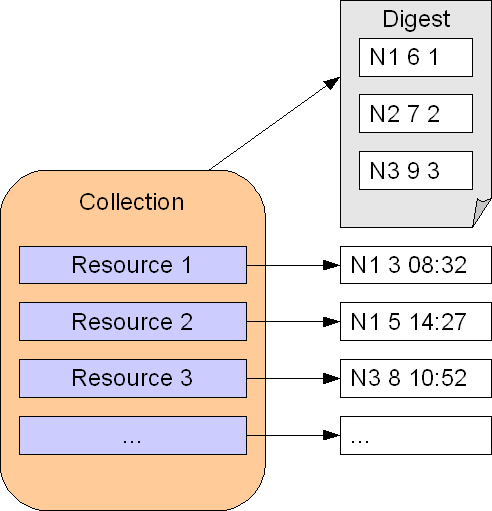# 2.4 Digest Variant

Usually we synchronize whole collections of resources rather than individual resources. So, SData uses a variant of the vector clock algorithm in which the nodes track the vector clocks at the collection level rather than at the resource level.

At the resource level, the SData algorithm only tracks the last modification: a single (Ni, Ti, Si) triplet where Ni is the node where the resource was last modified, Ti the tick at that node when the modification was detected and Si the modification timestamp (to solve conflicts when priorities are equal). This is less expensive and easier to implement because the synchronization metadata can be stored in a syncdata table with a simple one to one join to the resource table.

The collection level vector clock is called “digest” in the SData terminology. The SData digest is actually an “extended” vector clock made of triplets (node id, tick, conflict priority).

The following diagram shows how the sync metadata is organized at the collection and resource level:This variant of the vector clock algorithm assumes that synchronization is performed one collection at a time rather than one resource at a time (although it can also be used to propagate changes on individual resources, under certain conditions).

The digest of a node can also be interpreted as a “summary” of the vector clocks of all the resources of the collection at that node. For example, if the digest for a collection is (N1 6 1)(N2 7 2)(N3 9 3) at node N1, this means that N1’s collection has been synchronized with N2’s collection at tick 7 (N1’s state takes into account all changes made on N2 with tick < 7) and with N3’s collection at tick 9 (N1’s state takes into account all changes made on N3 with tick < 9)

Synchronization now relies on the following principles:

1. A collection may have different digests at different nodes. The goal of the synchronization system is to bring the collections in sync across nodes. When a collection is fully synchronized, its digest is the same on all nodes.

2. By comparing the digests of a given collection on two nodes, the synchronization algorithm can select the resources that need to be synchronized in both directions (see Change Selection with Digest).

3. The algorithm does not have complete vector clocks at the resource level but it has four pieces of information at hand when it synchronizes a resource: the two digests + the two resource level triplets. This is sufficient to detect and resolve conflicts (see Conflict Handling with Digest).